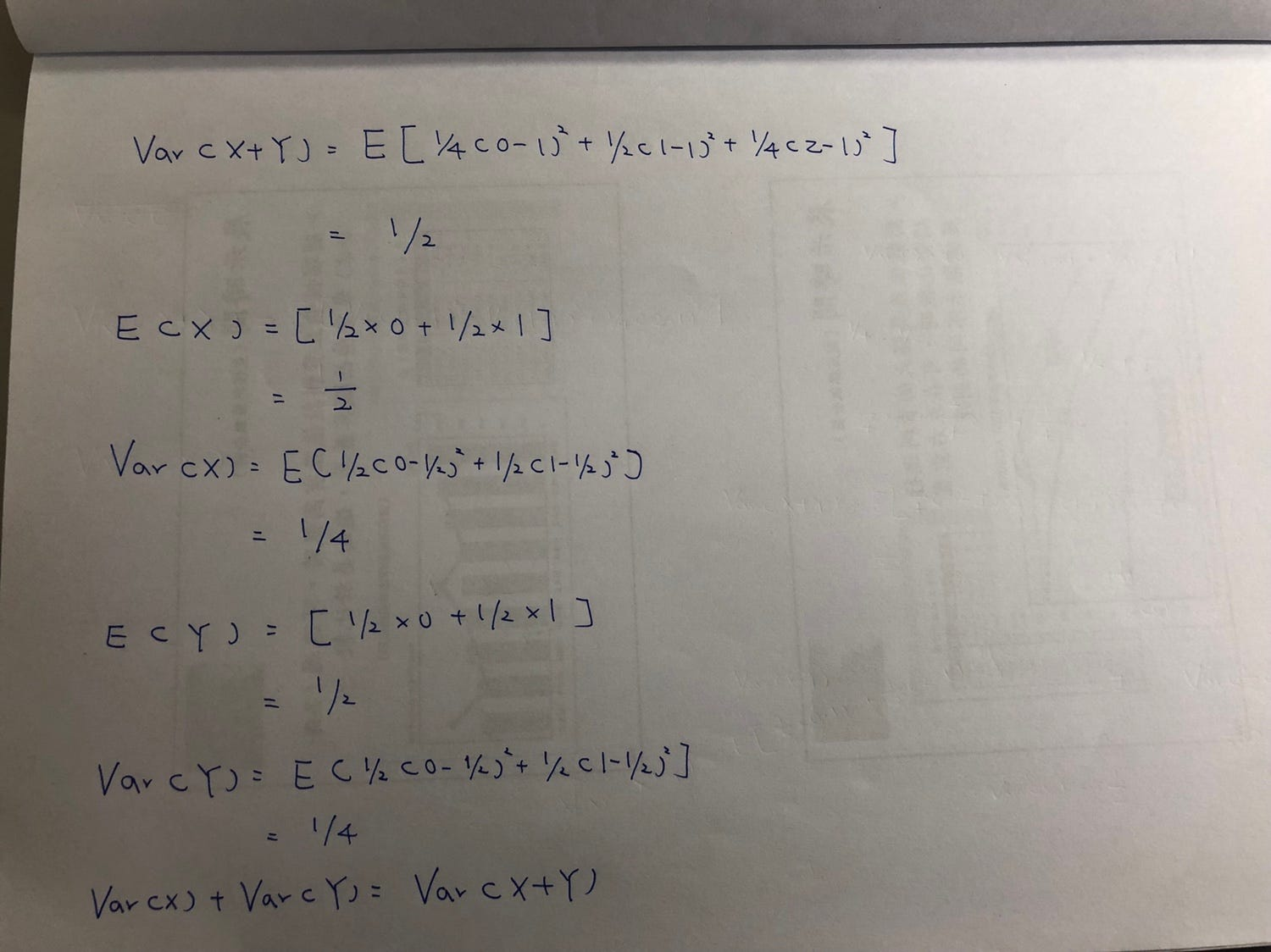# Let’s verify that Var(X+Y)=Var(X)+Var(Y) (two independent random variables)

Let’s define X and Y to each be the result of the coin flip where we assign the value 1 to Heads and 0 to Tails. So X+Y take on the values…Continue reading on Medium »